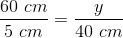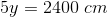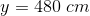# High School Math : Understanding Rational Expressions

## Example Questions

### Example Question #1 : Rational Expressions

Solve:

Ifvaries directly as, and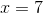when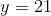, findwhen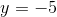.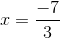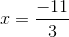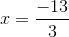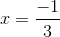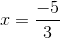Explanation:

The formula for a direct variation is: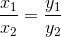Plugging in our values, we get: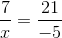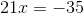### Example Question #2 : Rational Expressions

If two boxes have the same depth and capacity, the length is inversely proportional to the width. One box is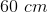long and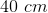wide. A second box (same depth and capacity) is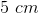long. How wide is it?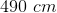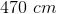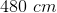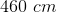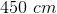Explanation:

The formula for an indirect variation is: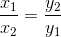Plugging in our values, we get: